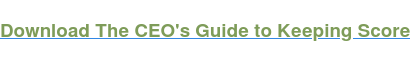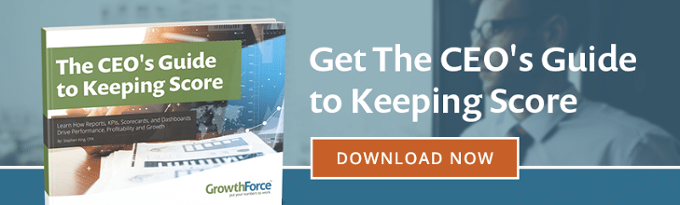# Convertible Debt Instrument (Bond)

A convertible bond is a debt instrument issued by a company that can be exchanged for shares of that company’s common stock. The price at which the bond can be converted into stock, or the conversion price, is typically set when the bond is issued. The bond can be converted at any point up until maturity.

Like standard nonconvertible bonds, issue a convertible bond with a set par value, maturity date, and coupon rate.

Convertibles are attractive instruments to investors because they combine the reliability of a debt instrument with the potential upside profits of shares of stock. If the company’s stock goes down, the convertible bondholder is not adversely affected. If the company’s stock goes up, the investor can profit from the conversion. The downside, however, is that a convertible bond will offer the investor a lower yield than a nonconvertible bond. This is because of the potential benefits of conversion feature. Convertible bonds are also typically callable, which means the issuing company can force the investor to convert the bond for a specified number of shares of stock at a certain price.

Issuing a convertible bond is an attractive financing option for a company because it is cheaper than issuing a nonconvertible bond. The benefits of the conversion feature allow the issuing company to pay a lower coupon rate to the bondholder. It is also a convenient way to raise funds without having to issue more common stock or standard debt instruments. On the downside, the convertible bond will dilute the company’s stock if converted.The convertible bond premium, or conversion premium, is the difference between the current stock price and the conversion price. For example, if a convertible bond can be exchanged for stock at \$50 per share, and the current stock price is \$45, then the conversion premium is \$5.

### Conversion Ratio Formula

The conversion ratio measures the number of shares of common stock the investor will receive in exchange for a convertible bond. The conversion ratio is often set at the issuance of the convertible instrument.

Conversion Ratio = Par Value of Convertible Bond / Conversion Price

For example, if the par value of the convertible bond is \$1,000 and the conversion price is \$50, then the conversion ratio equals 20. The investor could receive 20 shares of stock in exchange for each convertible bond.

### Conversion Value Formula

The conversion value of a convertible bond is the value of the instrument in terms of the underlying stock. If the conversion value is greater than the par value, then the investor earned a profit. If the conversion value is less than the par value, the investor has made a profit.

Conversion Value = Current Stock Price x Conversion Ratio

For example, if the par value is \$1,000, the conversion ratio is 20, and the stock price is \$40, then the conversion value is \$800. The investor would not want to convert. However, given the same par value and conversion ratio, if the stock price were \$55, then the conversion value would be \$1,100. In this case, the investor might want to convert the bond into stock and take the profit of \$100.